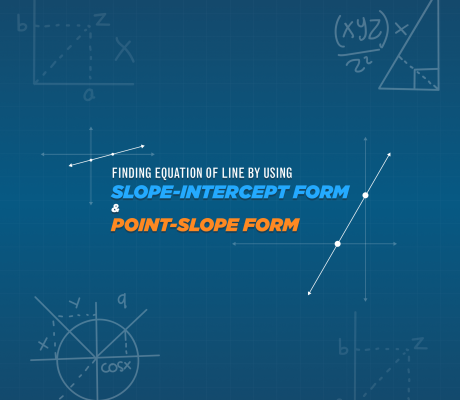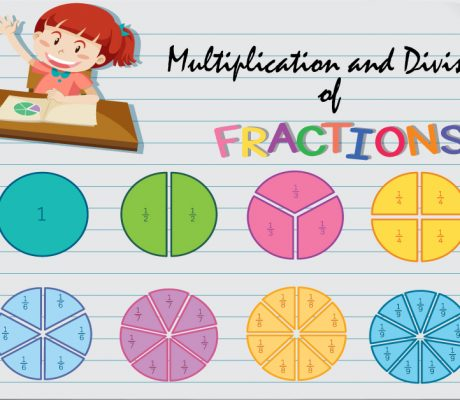# Category: Math Lessons Online### Finding The Equation Of The Line By Using The Slope-Intercept Form And Point-Slope Form

In mathematics and physics, equations of the line are used widely. Equation of the line is very essential in different branches of science and everyday problems. It is that equation in which the degree of each variable is one. This article will discuss the Slope-Intercept And Point-Slope of Linear Equations The equation of the line […]### Math Collaborative Learning

Some may consider online math education to be an individual venture. Imagine a student alone at their desk and trying to find all the answers on their own. With this kind of setup, it won’t help the students solve any problem. It may be the case for some teachers. Well, there are online institutions that […]### Equivalent Fractions

Equivalent fractions are fractions that represent the same size even if the numbers look different. Let’s take a look at the pizzas below. The two fractions have different numerators and denominators, but they represent the same size of pizza slices. when simplified, is actually . It is important to remember that these two fractions still […]### Multiplying and Dividing Fractions

The truth is multiplying and dividing fractions are easier than adding and subtracting fractions. It does not require a lot of steps because it only involves multiplying and simplifying.  When multiplying fractions, you take the numerators and multiply them. You also do the same with the denominators. If needed, the final product is then simplified […]